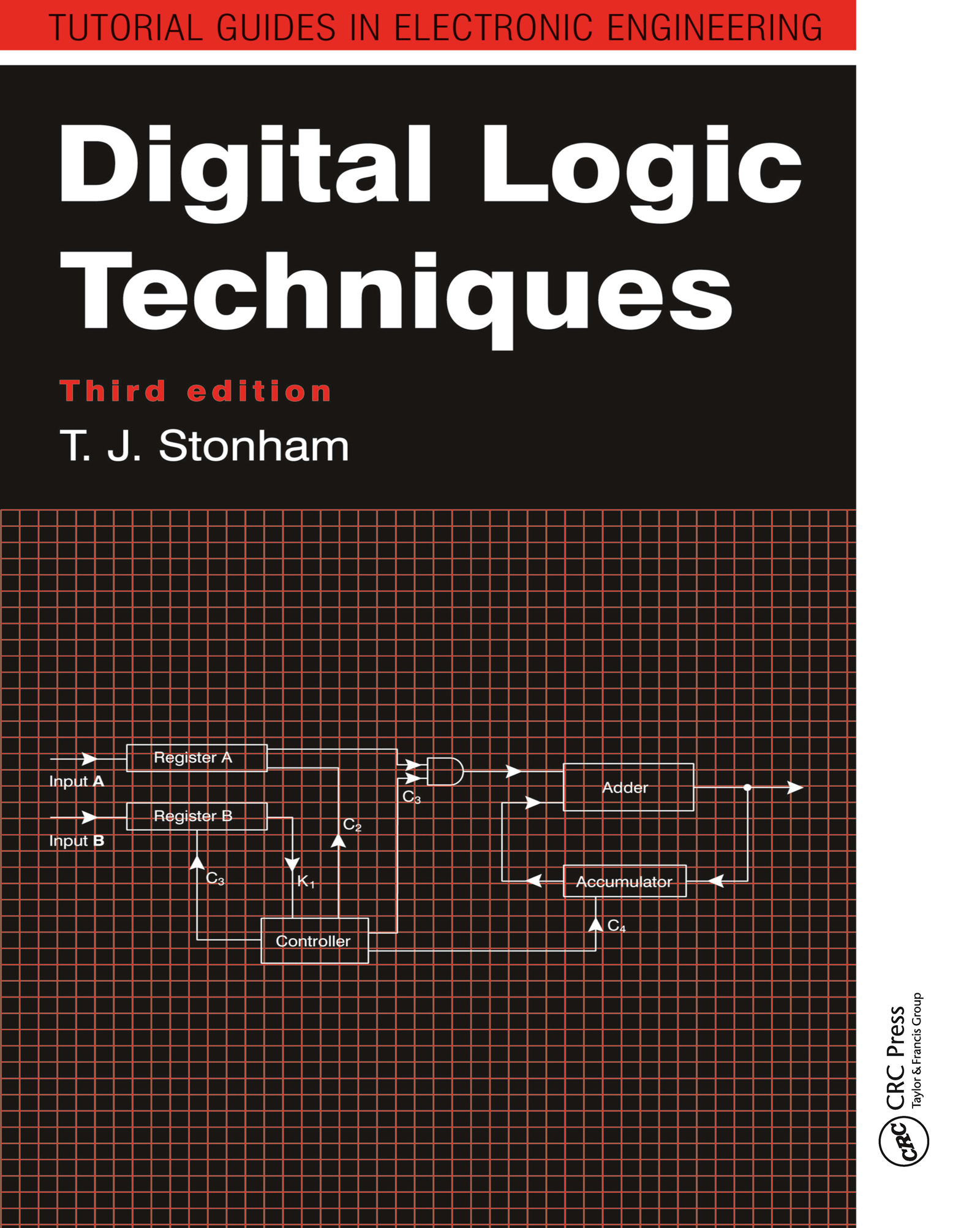# Digital Logic Techniques

## 3rd Edition

CRC Press

212 pages

Paperback: 9780748744497
pub: 1996-05-19
SAVE ~\$14.99
\$74.95
\$59.96
x
Hardback: 9781138410695
pub: 2017-10-02
SAVE ~\$41.00
\$205.00
\$164.00
x
eBook (VitalSource) : 9781315141305
pub: 2017-11-22
from \$37.48

FREE Standard Shipping!

### Description

The third edition of Digital Logic Techniques provides a clear and comprehensive treatment of the representation of data, operations on data, combinational logic design, sequential logic, computer architecture, and practical digital circuits. A wealth of exercises and worked examples in each chapter give students valuable experience in applying the concepts and techniques discussed.

Beginning with an objective comparison between analogue and digital representation of data, the author presents the Boolean algebra framework for digital electronics, develops combinational logic design from first principles, and presents cellular logic as an alternative structure more relevant than canonical forms to VLSI implementation. He then addresses sequential logic design and develops a strategy for designing finite state machines, giving students a solid foundation for more advanced studies in automata theory.

The second half of the book focuses on the digital system as an entity. Here the author examines the implementation of logic systems in programmable hardware, outlines the specification of a system, explores arithmetic processors, and elucidates fault diagnosis. The final chapter examines the electrical properties of logic components, compares the different logic families, and highlights the problems that can arise in constructing practical hardware systems.

NUMERICAL REPRESENTATION OF INFORMATION

Analog and digital data

Number systems

Conversion between Decimal and Binary Numbers

Non-Pure Binary Codes

Error Detecting Codes

Error Correcting Codes

Summary

Problems

OPERATIONS ON BINARY DATA

Logical Operations

Logic and Propositional Statements

Truth Tables

Binary Connectives

Logic Gates

Boolean Algebra

The Principle of Duality

Truth Table Equivalence

Summary

Problems

COMBINATIONAL LOGIC DESIGN

Assignment of Logic Levels

Specification of a Combinational Logic Circuit

The 1st Canonical Form

The 2nd Canonical Form

Conversion between Canonical Forms

Minimal Canonical Forms

The Karnaugh map

NAND Logic

NOT Logic

'Don't Care' Conditions

Hazards in Combinational Logic

Map-Entered Variables

Minimizing MEV Maps

Tabular method of Minimization

Prime Implicant Table

Cellular Logic

Summary

Problems

SEQUENTIAL LOGIC FUNDAMENTALS

A Sequential Logic Circuit

Limitations of the Set-Reset Flip-Flop

The JK Flip-Flop

The Synchronous Trigger Flip-Flop

The Delay Flip-Flop

Shift Registers

Summary

Problems

DESIGN OF SEQUENTIAL LOGIC CIRCUITS

The Parameters of a Sequential Circuit

Asynchronous Binary and Non-Binary Counters

Synchronous Sequential Logic

Cellular Sequential Logic

Autonomous Sequential Circuits

State Transition Diagrams

State Minimization in Sequential Logic

Equivalent States

The Implication Chart

Asynchronous Sequential Design

State Merging

State Assignment

The Asynchronous Nest State and Output Logic

Algorithmic State Machines (ASMs)

Summary

Problems

THE DIGITAL SYSTEM

Programmable Logic

Multilayer Multiplexer Systems

The Memory Element

Programmable Arrays of Logic (PALs)

Register Transfers

The Interconnection Problem

The Tristate Gate

The Bus Concept

Fault Diagnosis

The Fault Matrix

Boolean Difference Method

Path Sensitization

Fault Detection in Sequential Circuits

Fault-Tolerant Systems

Summary

Problems

DESIGN OF ARITHMETIC PROCESSORS

The Full Subtractor

Fast Subtractors

Signed Numbers

Subtraction Using Two's Complement Coding

Multiplication

Counter-Based Multipliers

Fast Multipliers

Parallel Multipliers

Look-Up Table Multipliers

Division

A General Arithmetic Processor

Design of the control Unit

Programming the System

Summary

Problems

PRACTICAL DIGITAL CIRCUITS

Logic Families

Bipolar Transistor Logic

Unipolar Logic Families

Practical Constraints on Logic Circuits

Interfacing CMOS and TTL

Practical Memory Circuits

Semi-Custom Logic

Interfacing the Digial System to the Real World

Problems Arising from Logic Usage

Summary

INDEX